Courses

# SSC CHSL Mock Test -14

## 100 Questions MCQ Test SSC CHSL Mock Test Series | SSC CHSL Mock Test -14

Description
This mock test of SSC CHSL Mock Test -14 for SSC helps you for every SSC entrance exam. This contains 100 Multiple Choice Questions for SSC SSC CHSL Mock Test -14 (mcq) to study with solutions a complete question bank. The solved questions answers in this SSC CHSL Mock Test -14 quiz give you a good mix of easy questions and tough questions. SSC students definitely take this SSC CHSL Mock Test -14 exercise for a better result in the exam. You can find other SSC CHSL Mock Test -14 extra questions, long questions & short questions for SSC on EduRev as well by searching above.
QUESTION: 1

Solution:

It is mentioned in the passage ' Businesses that engage in impact advertising are more likely to firmly establish themselves in the market because they create a lasting psychological impact on the audience, giving them valuable entertainment or information and creating a positive association with their product.'
Option 2 is the correct answer.

QUESTION: 2

Solution:

All the options except option 3 are mentioned in the passage. Advertisement cannot increase the price of any product.
Option 3 is thus the correct answer.

QUESTION: 3

Solution:

It is mentioned in the passage 'Businesses now face great competition in enhancing the influence of their advertising to create awareness, drive sales, maintain market share and establish brand identity. Advertisements inform customers of new offerings, reminds them of existing products and updates them on any modifications and changes that may increase their urge to buy.' Thus it is clear that advertisements are necessary for businesses to thrive.

Option 3 is thus the correct answer.
Option 2 is not mentioned in the passage.

QUESTION: 4

Q. Which of the following is MOST SIMILAR in meaning to the word 'jeopardy'?

Solution:

The word 'jeopardy' means 'danger'.
Deception means trickery.

Conspiracy means a secret plan by a group to do something unlawful or harmful.

Opposition means resistance or dissent, expressed in action or argument.
Thus option 1 is the correct answer.

QUESTION: 5

Q. Why are consumer protection laws needed?

Solution:

It is mentioned in the passage 'Consumer protection laws require businesses to refrain from false and misleading advertising.'

Option 3 is the correct answer.

QUESTION: 6

In the following question, out of the four alternatives, select the alternative that best expresses the meaning of the idiom/phrase.

At issue

Solution:

The correct answer is option 3, i.e., 'in dispute'.

The idiom 'at issue' means something that is under discussion or in dispute.

To understand the use of the phrase best, the idiom could be used in the sentence - The point at issue is quality.
Therefore, the correct answer is option 3.

QUESTION: 7

In the following question, out of the four alternatives, select the alternative that best expresses the meaning of the idiom/phrase.

In the long run

Solution:

The correct answer is option 1, i.e., 'eventually'.

The idiom 'in the long run' means over or after a long period of time; ultimately.

To understand the use of the phrase best, the idiom could be used in the sentence - Steady work is sure to be rewarded in the long run.
Therefore, the correct answer is option 1.

QUESTION: 8

In the following question, out of the four alternatives, select the alternative that best expresses the meaning of the idiom/phrase.

Put a spoke in one's wheel

Solution:

The correct answer is option 2, i.e., 'To thwart one in the execution of their design'.

The idiom 'put a spoke in one's wheel' means to disrupt, foil or cause problems to one's plan, activity, or project. The 'spoke' is used to refer to the problem that disrupts the plan. Just like a spoke can disrupt the movement of the wheel, similarly, these problems disrupt the plan or the project.

To understand the use of the phrase best, the idiom could be used in the sentence - I had everything in line for the party, but having the caterer cancel on me at the last minute really put a spoke in my wheel.
Therefore, the correct answer is option 2.

QUESTION: 9

In the following question, out of the four alternatives, select the alternative that best expresses the meaning of the idiom/phrase.

Flesh and blood

Solution:

The correct answer is option 1, i.e., 'human nature'.

The idiom 'flesh and blood' is used to lay stress on the fact that a person is a physical, living being with human emotions, often in contrast to something abstract, spiritual, or mechanical. In simple words, it represents the actual human nature.

To understand the use of the phrase best, the idiom could be used in the sentence - The customer is flesh and blood, not just a sales statistic.
Therefore, the correct answer is option 1.

QUESTION: 10

A sentence has been given in Active/Passive Voice. Out of the four alternatives suggested, select the one which best expresses the same sentence in Passive/Active Voice.

Somebody stole my purse.

Solution:

The sentence is present in the active voice. Sometimes, when the 'doer' or the subject of an action is not definite, like 'somebody' or 'nobody'; it is not necessary to use these words in the passive voice.

The passive voice gives importance to the action and not to the doer. So, here, there is no need to mention the indefinite 'doer', which is 'somebody'. So, the answer could be both 'my purse is stolen by somebody' and 'my purse is stolen'.
Therefore, the correct answer is option 2.

QUESTION: 11

A sentence has been given in Active/Passive Voice. Out of the four alternatives suggested, select the one which best expresses the same sentence in Passive/Active Voice.

The teacher told the students to go to the camp.

Solution:

The sentence is in the active voice. To turn it into passive, the subject and object, viz, 'teacher' and 'students' would exchange places. The phrase 'to go to the camp' would remain as it is.

The simple past tense verb 'told' would be adjusted to 'were'(as students is a plural noun)+ the past participle form of the verb, viz, 'told'.
Therefore, the correct answer is option 4.

QUESTION: 12

In the following question, a sentence has been given in Active/Passive voice. Out of the four alternatives suggested, select the one, which best expresses the same sentence in Passive/Active voice.

The tiger will have killed the animals

Solution:

Here, the basic structure of voice should be known to answer these type of questions.

The original sentence is in the future perfect tense. In such case the passive formation will be 'object + will/shall + have been + V3 + subject'.
Hence the correct answer is option 3.

QUESTION: 13

In the following question, a sentence is given in Direct/Indirect speech. Out of the four alternatives suggested, select the one which best express the same sentence in Direct/Indirect speech.

The students said “We will not be singing this song.’

Solution:

Here, the basic knowledge of direct and indirect speech is important to answer these type of questions correctly.

The original sentence is in direct speech. “Will + be + ing“ is used in the direct speech when it is in the future progressive tense. So we must change “will + be + ing” to “would + be + ing” in the indirect speech, and “this” must be changed to “that”.
Hence, option 3 is the correct answer.

QUESTION: 14

In the following question, a sentence is given in Direct/Indirect speech. Out of the four alternatives suggested, select the one which best express the same sentence in Direct/Indirect speech.

Rakhi said “I don’t play now."

Solution:

Here, the basic knowledge of direct and indirect speech is important to answer these type of questions correctly.

The original sentence is in the simple present tense in direct speech. So we must change the tense into simple past in the indirect speech. In the indirect speech “now” must be changed to “then”.
Hence, option 4 is the correct answer.

QUESTION: 15

In the following question, a sentence has been given in Direct/Indirect speech. Out of the four alternatives suggested, select the one which best express the same sentence in Indirect/Direct speech.

He said, “I bought an iPhone 6 yesterday.”

Solution:

The rule when converting simple past tense to reported speech is to add had+ bought. This is given by option 1.
Therefore, the correct answer is option 1.

QUESTION: 16

In the following question one part of the sentence may have an error. Find out which part of the sentence has an error and click the button corresponding too it. If the sentence is free from error, click the ''No error'' option.

Q. The principle thing (1)/ in this world is to (2)/ keep ones soul aloft (3) No error (4)

Solution:

Ones is just the plural of 'one.' This is not the correct word here. Here we must show possession when it talks about the soul. So we must use the apostrophe with 'one' to make it 'one's '

Correct Sentence: The principle thing in this world is to keep one's soul aloft.
Hence the correct answer is option 3.

QUESTION: 17

In the following question one part of the sentence may have an error. Find out which part of the sentence has an error and click the button corresponding too it. If the sentence is free from error, click the ''No error'' option.

Q. Keep your eyes (1)/ wide open before marriage, (2)/ and half shut afterward. (3) No error (4)

Solution:

There is no error in the sentence. The only possibility of error being in the word 'afterward.' But this word is correct. In fact, both 'afterward' and 'afterwards' are correct.
Hence the correct answer is option 4.

QUESTION: 18

In the following question one part of the sentence may have an error. Find out which part of the sentence has an error and click the button corresponding too it. If the sentence is free from error, click the ''No error'' option.

Q. Have Hindi (1)/ or English been (2)/ made compulsory? (3) No error (4)

Solution:

When two subjects are connected by 'or' we must use a singular verb. So here the correct verb is 'has.'
Correct Sentence: Has Hindi or English been made compulsory?
Hence the correct answer is option 1.

QUESTION: 19

In the following questions, some part of the sentence is underlined. Which of the options given below the sentence should replace the part underlined to make the sentence grammatically correct? If the sentence is correct as it is given then choose option 4 ‘No Correction required’ as the answer.

Q. You need courage to speak for your father's atrocities.

Solution:

The correct preposition is 'against' as a person would talk protesting against someone's atrocities. Since the sentence already has the pronoun 'you' the correct pronoun is 'your' and not 'one's.' Option 3 is thus the correct answer.

QUESTION: 20

In the following questions, some part of the sentence is underlined. Which of the options given below the sentence should replace the part underlined to make the sentence grammatically correct? If the sentence is correct as it is given then choose option 4 ‘No Correction required’ as the answer.

Q. The news of blasting up of the rocket in Russia created quite a buzz in the media.

Solution:

The correct phrasal verb is 'blasting off' which means 'a rocket or spaceship leaving the ground.' Option 3 is thus the correct answer.

QUESTION: 21

Complete the passage by choosing the correct words for the numbered blanks.

Banks in India as a whole were very __(A)__ to adopt the changes brought about by technological __(B)__ but a number of factors brought about the mechanization and digitization in the banking industry in India. The putting in place of standard cheque encoders was the first step forward in digital __(C)__ in banking. Magnetic Ink Character Recognition (MICR) helps in the __(D)__ and processing of cheques __(E)__ each bank branch having a MICR code.

Q. Which of the following fits in the blank labelled (A)?

Solution:

The word 'but' in the sentence makes it understood that banks were not open to adopting the technological changes but some factors brought about the changes. Thus 'reluctant' which means 'hesitant' fits here correctly. The other options cannot be placed here. 'Allured' means 'attracted.'

The complete sentence will be: Banks in India as a whole were very reluctant to adopt the changes brought about by technological
Option 4 is the correct answer.

QUESTION: 22

Complete the passage by choosing the correct words for the numbered blanks.

Banks in India as a whole were very __(A)__ to adopt the changes brought about by technological __(B)__ but a number of factors brought about the mechanization and digitization in the banking industry in India. The putting in place of standard cheque encoders was the first step forward in digital __(C)__ in banking. Magnetic Ink Character Recognition (MICR) helps in the __(D)__ and processing of cheques __(E)__ each bank branch having a MICR code.

Q. Which of the following fits in the blank labelled (B)?

Solution:

The passage talks about the progress of banks in the technological field thus option 1 fits here correctly. The other words do not convey any meaning here. The word 'disputes' means 'conflicts.'

The complete sentence will be: technological advancement but a number of factors brought about the mechanization and digitization in the banking industry in India.
Option 1 is the correct answer.

QUESTION: 23

Complete the passage by choosing the correct words for the numbered blanks.

Banks in India as a whole were very __(A)__ to adopt the changes brought about by technological __(B)__ but a number of factors brought about the mechanization and digitization in the banking industry in India. The putting in place of standard cheque encoders was the first step forward in digital __(C)__ in banking. Magnetic Ink Character Recognition (MICR) helps in the __(D)__ and processing of cheques __(E)__ each bank branch having a MICR code.

Q. Which of the following fits in the blank labelled (C)?

Solution:

The word 'transformation' means 'advancement' and fits here correctly. The sentence means that it was the first step of digital advancement. The other words cannot be placed here. 'Era' means 'a long and distinct period of history.'

The complete sentence will be: The putting in place standard cheque encoders was the first step forward in digital transformation in banking.
Option 2 is the correct answer.

QUESTION: 24

Complete the passage by choosing the correct words for the numbered blanks.

Banks in India as a whole were very __(A)__ to adopt the changes brought about by technological __(B)__ but a number of factors brought about the mechanization and digitization in the banking industry in India. The putting in place of standard cheque encoders was the first step forward in digital __(C)__ in banking. Magnetic Ink Character Recognition (MICR) helps in the __(D)__ and processing of cheques __(E)__ each bank branch having a MICR code.

Q. Which of the following fits in the blank labelled (D)?

Solution:

Banks would not print or collect cheques thus options 2 and 4 cannot be placed here. The word 'distribution' does not fit too. Only 'sorting' which means 'arranging in a particular way' fits here correctly.

The complete sentence will be: Magnetic Ink Character Recognition (MICR) helps in the sorting and processing of cheques
Option 3 is the correct answer.

QUESTION: 25

Complete the passage by choosing the correct words for the numbered blanks.

Banks in India as a whole were very __(A)__ to adopt the changes brought about by technological __(B)__ but a number of factors brought about the mechanization and digitization in the banking industry in India. The putting in place of standard cheque encoders was the first step forward in digital __(C)__ in banking. Magnetic Ink Character Recognition (MICR) helps in the __(D)__ and processing of cheques __(E)__ each bank branch having a MICR code.

Q. Which of the following fits in the blank labelled (E)?

Solution:

The correct preposition here is 'with' and not 'at' as the processing is linked with banks and does not take place necessarily at the physical location. The other prepositions do not fit here.

The complete sentence will be: processing of cheques with each bank branch having a MICR code.
Thus option 1 is the correct answer.

QUESTION: 26

If ‘+’ means ‘÷’, ‘×’ means ‘-’, ‘-’ means ‘+’ and ‘÷’ means ‘×’, then

16 - 5 × 2 + 4 ÷ 12 = ?

Solution:

Given equation:
16 - 5 × 2 + 4 ÷ 12 = ?
Equation after interchanging signs:
16 + 5 - 2 ÷ 4 × 12 = ?
⇒ 16 + 5 - 6 = ?
⇒ 15 = ?
Hence, ‘15’ is the correct alternative.

QUESTION: 27

In the following question, select the related word from the given alternatives.

Cope : Manage : : Correct : ?

Solution:

Cope and Manage have same meaning. Both Cope and Manage means to deal effectively with something difficult.

In the same way, Correct means to be free from errors and Right also means free from errors or exact.
Hence, Right is the correct answer.

QUESTION: 28

In a certain code language, “FRAME” is written as “IUDPH”. How is “ROYAL” written in that code language?

Solution:

F + 3 = I
R + 3 = U
A + 3 = D
M + 3 = P
E + 3 = H
Similarly,
R + 3 = U
O + 3 = R
Y + 3 = B
A + 3 = D
L + 3 = O
Hence, URBDO is the correct answer.

QUESTION: 29

In the following question, select the missing number from the given series.

19, 21, 25, 27, 31, ?

Solution:

The pattern followed here is,
19 + 2 = 21;
21 + 4 = 25;
25 + 2 = 27;
27 + 4 = 31;
31 + 2 = 33
Hence, 33 is the correct asnwer.

QUESTION: 30

If EAR = 24 and EYE = 35, then LEG = ?

Solution:

With reference to English alphabet, adding the numerical positions of each letter together would give the sum on the right hand of the equation.

To illustrate,
E + A + R = 24
( 5 + 1 + 18 = 24)
E + Y + E = 35
(5 + 25 + 5 = 35)
Similarly,
L + E + G = 24
(12 + 5 + 7 = 24)
Hence, ‘24’ is the correct answer.

QUESTION: 31

Read the statements and identify the logically correct conclusion from the given information.

Statement:
This scale is transparent.

Conclusions:
I. The scale is made up of glass.
II. The scale is made up of plastic.

Solution:

The statements say that, “This scale is transparent”. There is no information is given about the material of the scale. Transparency is a property which can be present in number of materials.

Hence, “Neither conclusion I nor II” can be concluded.

QUESTION: 32

Find the missing number of the series.

2, 0, 3, 5, 25, 119, ?

Solution:

Here the logic followed is:
2 × 1 – 2 = 0
0 × 2 + 3 = 3
3 × 3 - 4 = 5
5 × 4 + 5 = 25
25 × 5 – 6 = 119
119 × 6 + 7 = 721
Hence, 721 is the correct answer.

QUESTION: 33

From the given answer figures, select the one in which the question figure is hidden/embedded.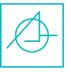Solution:

On close observation, we find that the problem figure is embedded in figure (3) as shown below,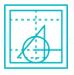Hence, figure 3 is the correct answer.

QUESTION: 34

In the following question, select the word which can be formed using the letters of the given word.

SIGNATURE

Solution:

Let’s check each option,
1) MAGENTA → cannot be formed as there is only one ‘A’ and no ‘M’ in SIGNATURE.
2) STRIP → cannot be formed as there is no ‘P’ in SIGNATURE.
3) STRANGE → can be formed as SIGNATURE.
4) SUGARY → cannot be formed as there is no ‘Y’ in SIGNATURE.
Hence, only ‘STRANGE’ can be formed from word SIGNATURE.

QUESTION: 35

A series is given with one term missing. Select the correct alternative from the given ones that will complete the series.

K, O, S, W, ?

Solution:

The pattern followed here is: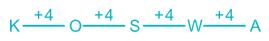QUESTION: 36

In the following question, a group of interrelated words is given. Choose a word from the given alternatives, which is similar to the given words and hence belongs to the same group.

Diet : Sansad : Majlis : ?

Solution:

Diet (of Japan), Sansad (of India), and Majlis (of Iran) are the names of parliaments of different countries.
Similarly,
Knesset is also a parliament name of Israel.

QUESTION: 37

Pointing to a photograph a lady said, “He is the son of my grandfather’s only son”. How is the person in the photo related to the lady?

Solution:

Lady’s grandfather’s son would be her father. The man in the picture is the son of her father. Therefore, the man in the picture is her brother.
Hence, ‘Brother’ is the correct answer.

QUESTION: 38

Direction: Arrange the given words in the sequence in which they occur in the dictionary.
1) Intend
2) Interest
3) Interfere
4) Internal
5) Integral

Solution:

On arranging given word as per dictionary order,
5) Integral
1) Intend
2) Interest
3) Interfere
4) Internal
Hence, “5, 1, 2, 3, 4” is the correct arrangement as per they occur in the dictionary.

QUESTION: 39

In the question two statements are given, followed by two conclusions, I and II. You have to consider the statements to be true even if it seems to be at variance from commonly known facts. You have to decide which of the given conclusions, if any, follows from the given statements.

Statement I: No bombs are mortar.
Statement II: All rockets are bombs.

Conclusion I: All mortar are rockets.
Conclusion II: Some bombs are rockets.

Solution:

The least possible Venn diagram for the given statements is as follows,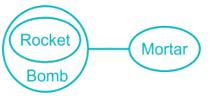Conclusions:
I. All mortar are rockets → False (It is not possible)
II. Some bombs are rockets → True (as shown in the above figure)
Hence, only conclusion II follows.

QUESTION: 40

Choose the odd one out from the given alternatives.

Solution:

All except Diamond are the elements in group table, whereas Diamond is a compound.

QUESTION: 41

Which answer figure will complete the pattern in the question figure?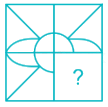Solution: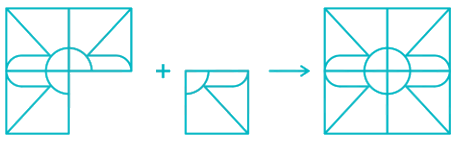Thus image at option 4 completes the given figure.

QUESTION: 42

How many rectangles are there in the given figure?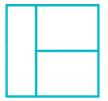Solution:

The rectangles formed in the given figure are as follows,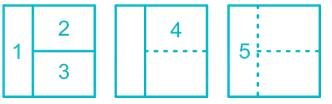Thus 5 rectangles are formed.

QUESTION: 43

Identify the diagram that best represents the relationship among the given classes.

Mother, Grandmother, Females

Solution:

As, some mothers can be grandmother and both mother and grandmother are female.
Therefore, it can be best shown as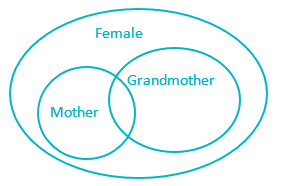Hence, option (1) is the correct answer.

QUESTION: 44

A piece of paper is folded and punched as shown below in the question figures. From the given answer figures, indicate how it will appear when opened?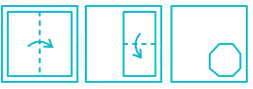Solution:Hence, the unfolded figure will be similar to option 1.

QUESTION: 45

Two position of a cube are shown below. What will come opposite to face containing ‘1’ ?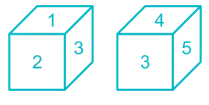Solution:

If we rotate first dice such that, 2 comes at bottom, then the position of both dices would be as shown below,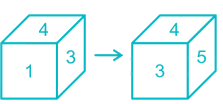From the above diagram, we can say that 2 is opposite to 4 and 1 is opposite to 5.
Hence, 5 is the correct answer.

QUESTION: 46

Which one of the given responses would be a meaningful order of the following?

1. Addition, 2. Multiplication, 3. Brackets, 4. Subtraction, 5. Division, 6. Orders

Solution:

The logical sequence (for BODMAS rule) will be as follows:

3. Brackets > 6. Orders > 5. Division > 2. Multiplication > 1. Addition > 4. Subtraction
Hence, ‘3, 6, 5, 2, 1, 4’ is the meaningful order.

QUESTION: 47

In the following question, select the odd word from the given alternatives.

Solution:

Here, pupil is a person who is taught by others whereas the supervisor, mentor and preceptor are all instructors.

QUESTION: 48

Choose the mirror image of the following figure.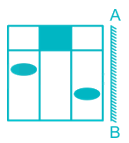Solution:

The image is flipped horizontally.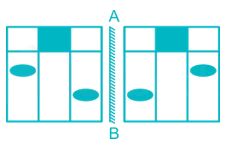Hence, option 4 is the mirror image of the given image.

QUESTION: 49

In the following question, four number pairs are given. The number on left side of (–) is related to the number on the right side of (–) with some Logic/Rule/Relation. Three are similar on basis of same Logic/Rule/Relation. Select the odd one out from the given options.

Solution:

17 × 2 = 34
19 × 2 = 38
23 × 2 = 46
27 × 2 = 54 ≠ 56
Hence, “27 – 56” is the correct answer.

QUESTION: 50

Sum of present ages of A and B is 47 years. After 5 years, there ages will be in ratio 8 : 11. What is the present age (in years) of A?

Solution:

Let x years be the present age of A & y years be the present age of B.
Sum of present ages = 47 → x + y = 47       …. Equation (1)
Then, After 5 years
Age of A = x + 5
Age of B = y + 5
Ratio=x + 5/y + 5= 8/11
By solving it we get:
11x - 8y = -15       …… Equation (2)
By solving equation (1) and equation second we get:
x = 19 & y = 28.
Hence, the present age of A is 19 years.

QUESTION: 51

A purse has Rs. 34.5 in the form of 1-rupee, 50-paise and 10-paise coins in the ratio of 6 ∶ 9 ∶ 10. Find the number of 10-paise coins.

Solution:

Let total coins in the purse are x.
⇒ 1-rupee, 50-paise and 10-paise coins would be 6x, 9x and 10x respectively.
Total amount is Rs. 34.5
⇒ 6x × 1 + 9x × 0.5 + 10x × 0.1 = 34.5
⇒ 6x + 4.5x + x = 34.5
⇒ 11.5x = 34.5
⇒ x = 3
∴ Number of 10-paise coins = 10 × 3 = 30

QUESTION: 52

The ratio between the present ages of Sonu and Monu is 3 : 4 respectively. Four years hence Monu will be 5 years older than Sonu. What is Sonu’s present age?

Solution:

⇒ Let ratio of Sonu and Monu present ages be 3x and 4x respectively
⇒ Four years hence, Sonu age = (3x + 4) years and Monu age = (4x + 4) years
⇒ According to question
⇒ 3x + 4 + 5 = 4x + 4
∴ x = 5
∴ Present age of Sonu = 3 x 5 = 15 years

QUESTION: 53

The third proportional of two numbers 4 and 28 is _______.

Solution:

The third proportional of two numbers a and b is defined to be that number c such that a : b = b : c.
Let c be the third proportion of 4 and 28
4 : 28 = 28 : c
c = (28 × 28)/4 = 196

QUESTION: 54

A point A south to the tower makes an angle of elevation of 30° and point B which is in the east makes an angle of 45° with the tower. If AB = 16 meter, then height to the tower is -

Solution: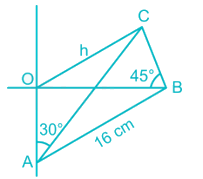Let OC be the tower. Suppose OC = h
In ΔOBC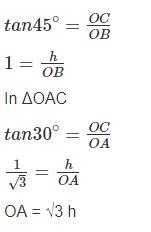In ΔOAB
AB2 = OA2 + OB2
(16)2 = (√3 h)2 + (h)2
AB2 = 3h+ h2
4h2 = 16 ×16
h = 8 meter

QUESTION: 55

Jugnu, a dishonest milk seller has certain quantity of milk to sell. Find the approximate ratio he should mix water to gain 5% by selling the mixture at the cost price?

Solution:

Let the cost price of 100 g milk was 100 units.
Let’s assume that x grams of milk are mixed with (100 – x) grams of water.
x + 5% of x = 100
⇒ x + 0.05x = 100
⇒ 1.05x = 100
⇒ x = 100/1.05 = 2000/21
Water = 100 – (2000/21) = 100/21
Ratio of water and Milk = 100/2000 = 1 : 20

QUESTION: 56

The ratio of spirit and water in the two vessels is 5 : 1 and 3 : 7 respectively. In what ratio the liquid of both the vessels should be mixed such that a new mixture containing half spirit and half water is obtained?

Solution:

Let x litre of first mixture and y litre of the second mixture are mixed.
Quantity of milk in x litre of first mixture = 5x/6
Quantity of milk in y litre of second mixture = 3y/10
Total quantity of the resultant mixture = (x + y)
Quantity of milk in (x + y) litre of the resultant mixture = (x + y) /2
5x/6 + 3y/10 = (x + y) /2
⇒ (25x + 9y) /30 = (x + y) /2
⇒ 25x + 9y = 15 × (x + y)
⇒ 25x + 9y = 15x + 15y
⇒ 10x = 6y
⇒ x/y = 3/5
∴ required ratio = 3 : 5

Alternative method :
Concentration of spirit in the first mixture = 5/6
Concentration of spirit in the second mixture = 3/10
Concentration of spirit in the resultant mixture = 1/2
By rule of allegation,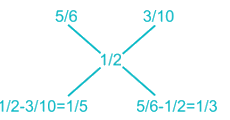∴ Required ratio = (1/5) : (1/3) = 3 : 5

QUESTION: 57

Determine the unit digit in the expansion of the following: (4987)184

Solution:

The given number is (4987)184
⇒ the unit digit of the given number will be the unit digit of number raised to 184 i.e., 7184
⇒ 71 = 7, 72 = 49 (unit digit is 9); 73 = 343 (unit digit is 3); 74 = 2401(unit digit 1)
⇒ 75 = 16807 (unit digit 7 again)
∴ with 7 as the unit digit raised to power, the only possibilities of unit digits are 7, 9, 3 and 1.
⇒ 7184 can be written as 7(4 × 46)
⇒ (74)46 ⇒ (1)46 = 1
∴ (4987)184 will have 1 as its unit digit.

QUESTION: 58

Find the (approx.) difference between the compound interest and the simple interest on Rs. 16,000 at 10% p.a. for 6 years.

Solution:

According to the given information, P = 16,000; R = 10%; T = 6

We know that the Simple interest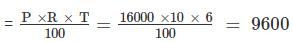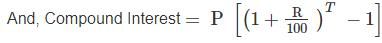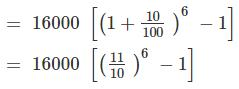= 16000 × 0.77 = 12320
Thus, the difference between the compound interest and the simple interest
= 12320 – 9600 = 2720
Hence, the difference is approximately Rs. 2720

QUESTION: 59

A certain distance is covered at a certain speed. If half of this distance is covered in double the time, the ratio of the two speeds is

Solution:

We know that Speed = Distance/Time

Case 1:
Let 100 kms be covered in 1 hour
Hence, speed = 100 kmph

Case 2:
Distance = 100/2 = 50 km
Time = 2 hours
Speed = 50/2 = 25 kmph
Required ratio = 100 kmph : 25 kmph
= 4 : 1

QUESTION: 60

A swimmer can cover 14 km in 2 hours, swimming in the direction of stream which is flowing at 3 km/hr. How much time will he take to cover the same distance against the stream?

Solution:

⇒ Downstream speed = 7 km/h
⇒ Speed of stream = 3 km/h
Speed of stream = 1/2 (Downstream speed – Upstream speed)
⇒ 3 = ½ (7 – Upstream speed)
⇒ Upstream speed = 7 – 6 = 1 km/h
∴ Time taken during upstream = 14/1 = 14 hours

QUESTION: 61

A moving train crosses a man standing on a platform and a bridge of 240 m in 5 s and 13 s respectively. What will be the time taken by the train to cross a platform 30 m long?

Solution:

Let the length of the train be x m.

A moving train crosses a man standing on a platform and a bridge 240 m in 5 s and 13 s respectively.
So, we can write now,
(x/5) = (x + 240)/13
⇒ 13x = 5x + 1200
⇒ 8x = 1200
⇒ x = 150

So, the speed of the train = 150/5 m/s = 30 m/s.

To cross a 30 m long platform, the train have to cover distance = 30 + 150 = 180 m.
∴ The time taken by the train to cross a platform 30 m long = (180/30) m/s = 6 s.

QUESTION: 62

At a telephone exchange, three phones ring at intervals of 20 sec, 24 sec and 30 seconds - If they ring together at 11:25 am, when will they next ring together?

Solution:

Time taken by three phones to ring together = LCM of 20, 24 and 30

⇒ Time taken by three phones to ring together = 120 seconds
(∵ 1 min = 60 seconds)

⇒ Time taken by three phones to ring together = 120/60 = 2 min

If the three phones ring together at 11:25 am then they will next ring together after 2 minutes i.e. at 11:27 am.
∴ Time at which the phones next ring together = 11:27 am

QUESTION: 63

Two taps A and B fill a tank in 24 minutes and 32 minutes respectively. If both the taps are opened simultaneously, after how many minutes tap B should be closed so that the tank fills up in 18 minutes.

Solution:

Tap A fills 1/24 of the tank in 1 minute
Tap B fills 1/32 of the tank in 1 minute

Let,
After x minutes tap B should be closed

so,
⇒ 18/24 + x/32 = 1
⇒ x/32 = 1 - 18/24
x = 6/24 × 32 = 8minutes

QUESTION: 64

A chord of length 60 cm is at a distance of 16 cm from the centre of a circle. What is the radius (in cm) of the circle?

Solution:

Let the chord be AB and O be the centre of the circle
The perpendicular bisector on AB be OC.
Now, ∆AOC is a right angled triangle.
So, AO2 = OC2 + AC2
⇒ AO2 = 162 + 302
⇒ AO = 34 cm
⇒ Radius = AO = 34 cm
∴ the correct option is 2)

QUESTION: 65

A cube of side 10 cm is painted on all its side. If it is sliced into 1 cc cubes, how many 1 cc cubes will have exactly one of their sides painted?

Solution:

When a 10 cc cube is sliced into 1 cc cubes, we will get 10 × 10 × 10 = 1000, 1 cc cubes

In each side of the larger cube, the smaller cubes on the edges will have more than one of their sides painted. Therefore, the cubes which are not on the edge of the larger cube and that lie on the facing sides of the larger cube will have exactly one side painted.

In each face of the larger cube, there will be 10 × 10 = 100 cubes. Of these, there will be 36 cubes on the edge and 8 × 8 = 64 cubes which are not on the edge.

Therefore there will be 64, 1 cc cubes per face that will have exactly one of their sides painted.

In total there are 6 faces and so 64 × 6 = 384 cubes with one side painted.

QUESTION: 66

A wall 15 m long, 4 m high and 60 cm thick is to be constructed. If the bricks have the dimension 24 cm × 10 cm × 8 cm, how many bricks are required to construct the wall?

Solution:

Volume of wall = 1500 cm × 400 cm × 60 cm = 36 × 106 cm3           [∵ 1 m = 100 cm]
Volume of one brick = 24 cm × 10 cm × 8 cm = 1920 cm3
Number of bricks required = (Volume of wall)/(Volume of one brick)
∴ Number of bricks required = (36 × 106)/1920 = 18750

QUESTION: 67

Two tangents are drawn from a point C to a circle at A and B. O is the centre of the circle. If ∠AOC = 60°, then ∠ACB is

Solution: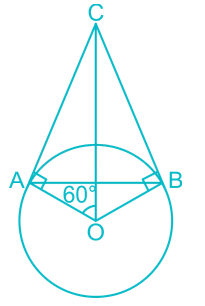⇒ IN ΔAOC
⇒ ∠AOC = 60° (Given)
⇒ ∠CAO = 90°
∴ ∠OCA = 180°- ( 90° + 60°) = 30°
⇒ ∠ACB = 2 × ∠OCA = 2 × 30° = 60°

QUESTION: 68

A chord having a length 24 cm is at a distance of 16 cm from the centre of a circle. The radius of the circle is -

Solution: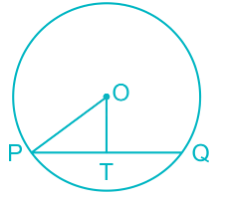The length of a chord of a circle is 24 cm and is at a distance of 16 cm from the centre of the circle.
From the figure,
∠PTO = 90° and PT = TQ = 24/2 = 12 cm and OT = 16 cm.
In Δ PTO,
OP2 = PT2 + OT2
= 122 + 162 = 400
Then, OP = √400 = 20
Hence, the radius of the circle = 20 cm.

QUESTION: 69

If the sum of the interior angles of the polygon is 1710°, find the number of sides of the polygon.

Solution:

Sum of interior angles of the polygon = 180 (n - 2) where ‘n’ is the number of sides of the polygon
⇒ 180 (n - 2) = 1710°
⇒ (n - 2) = 1710/180
⇒ (n - 2) = 9.5
⇒ n = 11.5
Number of sides of the polygon cannot be a decimal number
∴ None of these is the right option

QUESTION: 70

Five men or ten women can complete a job in 20 days. In how many days can 3 men and 4 women complete it?

Solution:

Five men or ten women can complete a job in 20 days,
⇒ m = 2w
⇒ Total work = 5 × 20 = 100 units
⇒ 3 men and 4 women = 3m + 4 × (m/2) = 5m
⇒ 3 men and 4 women can complete work in 100/5 = 20 days

QUESTION: 71

What is the net discount for successive discounts of 30% and 40%?

Solution:

Short trick:
Net successive discount = 30% + 40% - [(30 × 40)/100] = 58%

Detailed solution:
Let the market price be Rs.100.
Net successive discounts = (30/100) × 100 = 30
New market price = 100 - 30 = Rs.70
Price after 40% discount = (40/100) × 70 = 28
Net price after two discounts = 70 - 28 = Rs.42
∴ required discount = 100 - 42 = 58%

QUESTION: 72

ΔUVW is right angled at V. If sec U = 5/3, then what is the value of tan W?

Solution:

Given, ΔUVW is right angled at V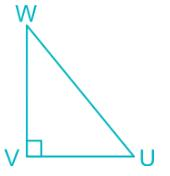sec U = 5/3,
⇒ Hypotenuse/Base = 5/3
⇒ UW/VU = 5/3
Let UW = 5k and UV = 3k
In Δ VUW
⇒ (UW)2 = (VW)2 + (VU)2
⇒ (5k)2 = (VW)+ (3k)2
⇒ VW = 4k
∴ tan W = Perpendicular/base = UV/VW = 3k/4k = 3/4

QUESTION: 73

Direction: The bar graph shows the population of different countries. Study the diagram and answer the following questions.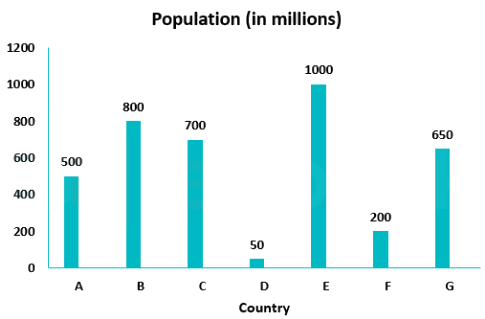Q. Population of country E is greater than that of country A by ___________.

Solution:

Population of country E = 1000
Population of country A = 500
Difference in the population of E and A = 1000 - 500 = 500

Population of country E is greater than that country A by [(Difference of the population)/Population of country A] × 100%
⇒ [500/500] × 100% = 100%
∴ Required Percentage = 100%

QUESTION: 74

Direction: The bar graph shows the population of different countries. Study the diagram and answer the following questions.Q. What is the ratio of population of country A to that of country G?

Solution:

Population of country A = 500
Population of country G = 650
Ratio of population of country A to country G = 500 : 650 = 10 : 13
∴ Required Ratio = 10 : 13

QUESTION: 75

Direction: The bar graph shows the population of different countries. Study the diagram and answer the following questions.Q. Which country has the second highest population?

Solution:

From the graph, it can be seen that the second highest value is for country B which is 800
So, the country with the second highest population is 800

QUESTION: 76

Who was the first president of China?

Solution:
• Sun Yat-sen was the first president of China.
• He is referred as the "Father of the Nation" in the Republic of China due to his instrumental role in the overthrow of the Qing dynasty during the Xinhai Revolution.
• Sun remains a unique figure among 20th-century Chinese leaders for being widely revered in both mainland China and Taiwan.
QUESTION: 77

When is National Girl Child day celebrated?

Solution:

National girl child day is celebrated every year on 24th of January as a national observance day for the girl child. It is to offer more support and new opportunities for the girls in the country. It aims to increase awareness among people about all the inequalities faced by the girl child in the society.
Note: 11 October - International Girl Child Day

QUESTION: 78

Who is the first Chief Minister of Kerela?

Solution:

On 1 November 1956, the States Reorganisation Act redrew India's map along linguistic lines state of Kerala was born, consisting solely of Malayalam-speaking districts. The first was E. M. S. Namboodiripad of the Communist Party of India, whose tenure was cut short by the imposition of President's rule.

QUESTION: 79

Kandyan is a dance form of which country?

Solution:
• Kandyan  is a dance form of Sri Lanka.
• Originally only males were allowed train as dancers, there are now several schools which also train women in the Kandyan dance form.
QUESTION: 80

Who is/was the longest serving Prime Minister of Bangladesh?

Solution:

​Sheikh Hasina is the longest serving Prime Minister of Bangladesh.

QUESTION: 81

Who has become the youngest ever Lok Sabha MP?

Solution:
• BJD’s Chandrani Murmu became the youngest ever Lok Sabha MP.
• The 25-year-old BTech graduate won the reserved Keonjhar, Odisha's seat by defeating a two-time MP.
• The Keonjhar Lok Sabha seat is reserved for Scheduled Tribes.
QUESTION: 82

Who is the first woman fighter pilot to fly by day on a Hawk advanced jet aircraft?

Solution:
• Flight Lt Mohana Singh has become the first woman fighter pilot to fly by day on a Hawk advanced jet aircraft.
• Her training involved flying both air-to-air combat and air-to-ground missions.
• She and two other women officers, Bhawana Kanth and Avani Chaturvedi, joined the fighter stream in June 2016.
• Flight Lt Bhawana Kanth became the first daytime woman fighter pilot to fly a MiG-21 Bison.
QUESTION: 83

The First member of homologous series of alkene is

Solution:

Homologous Series:

• A series of compounds in which the same functional group substitutes for hydrogen in a carbon chain is called a homologous series
• Successive terms differ by one functional unit

Alkenes

• Alkenes contain double bond between 2 carbon atoms and hence minimum two carbon atoms are required to form an alkene
• For alkenes, first member is ethene followed by propene, butene etc.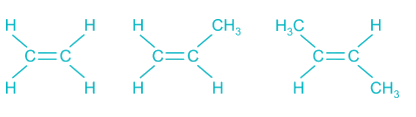QUESTION: 84

Who plundered Somnath Temple?

Solution:
• Sultan Mahmud of Ghazni attacked India 17 times in order to plunder the wealth from the country.
• It was the first Turkish invasion in the country.
• He raided the Somnath Temple on the coast of Kathiawad in the year 1025 AD and the temple was destroyed in the year 1026 AD.
QUESTION: 85

Who is the founder of Facebook?

Solution:

Mark Zuckerberg is the founder of Facebook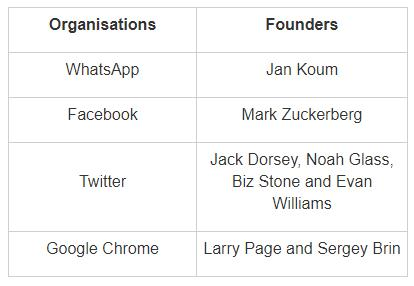QUESTION: 86

Which among the following is the capital of Estonia?

Solution:

Tallinn is the capital of Estonia.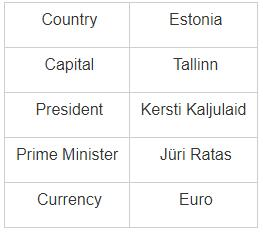QUESTION: 87

Who among the following is the South African President ?

Solution:
• African National Congress (ANC) won South African elections with 57.51% votes and Cyril Ramaphosa elected as South African President.
• Last year in February 2018, Cyril Ramaphosa was elected as South African President after President Jacob Zuma resigned from the post.
• It is relevant as India has close ties with South Africa.Both are part of IBSA  and have historic ties.
QUESTION: 88

Who among the following built the Shore Temple?

Solution:
• Shore Temple was built by Narasimhavarman-II.
• It was built in the 8th century in Mahabalipuram (Tamil Nadu).
• Anand Bhavan was built by Motilal Nehru in 1930 AD.
• Rock Cut Temple was built by Mahendravarman-I in the 8th Century.
• Nalanda University was built by Kumaragupta-in the 5th Century.
QUESTION: 89

Where are the Sebaceous glands located?

Solution:
• Sebaceous Glands are located in the Skin.
• They secrete an oily or waxy matter, called sebum.
• They occur on all parts of the skin except sole of the feet and palm of the hands.
• Salivary Glands are located in the Mouth.
• Gastric Glands, Pyloric Glands, and Parietal Cell are located in the Stomach.
• Submandibular Glands are located in the Tongue.
QUESTION: 90

What is the radius of the Earth at the equator?

Solution:
• The Earth’s radius at the equator is the 6378 km.
• Earth's polar radius is 3, 950 miles  (6, 356 km) which is about 22 km more than that of the radius at Equators.
• According to NASA, it is the densest planet in the solar system because of its metallic core and rocky mantle.
• The mean radium is 6371 Km.
QUESTION: 91

Which among the following was India’s first indigenously built submarine?

Solution:
• INS Shalki was India’s first indigenously built submarine.
• It was a Shishumar-class diesel-electric submarine of the Indian Navy.
• It was the first ever submarine to be built in India.
• It was launched in 1987 by the late Prime Minister Rajiv Gandhi.
• INS Vibhuti was India’s first indigenously built missile boat launched at Mazagon Dock Shipbuilders Ltd.
• INS Savitri was India’s first fabricated at Hindustan Shipyard Limited in 1990.
• INS Chakra was a type of Nuclear-powered attack submarine.
QUESTION: 92

Which is the smallest bird in the world?

Solution:

The Hummingbird is the smallest bird on the planet, just weighing at 1.6 grams with some males measuring only 57 mm in length. They are natives of Cuba and The Isle of Youth.

QUESTION: 93

Why Taj Mahal is suffering from "Marble Cancer"?

Solution:
• Marble cancer refers to the corrosion of structure made up of marble by the action of sulphuric or nitric acid.
• In the last decade, there was huge industrial growth nearby Taj Mahal, and the huge amount of sulfur and nitric gases are released in the atmosphere by industries.
• These gases mixed with rain cause acid rain. And The Taj Mahal is losing its white shine because of marble cancer.
QUESTION: 94

Which article of the Indian Constitution contains the procedure for Impeachment of President?

Solution:
• The Article 61 of the Indian Constitution contains the procedure for Impeachment of President.
• The procedure of Impeachment of President of India can be initiated only on the grounds of Violation of Constitution.
• No President has been impeached till date in India.
QUESTION: 95

G20 ministerial meeting is being held in which country?

Solution:

The 1st G20 meeting of Finance Ministers and Central Bank governors began on 19th March in Buenos Aires, with the presence of 57 delegations from countries and institutions. The topics under discussion are likely to be infrastructure and the future of work in the digital arena. This is the first of five such meetings to be held before Argentina hosts the G20 Summit.

QUESTION: 96

Largest river island in the India is _________.

Solution:

Majuli is a large river island in the Brahmaputra River, in Assam. Majuli is the largest river island in the world. Majuli had a total area of 1,250 square kilometres, but due to erosion now it has an area of only 421.65 square kilometres in 2001.

QUESTION: 97

Who wrote “Geet Govind”?

Solution:

It was Jaidev who wrote Geet Govind. It’s a 12th century work, which describes the relationship between Krishna and gopis of vrindavan and in particular one gopi named Radha.

QUESTION: 98

Who was the first Chief Justice of India?

Solution:

Sir H. J. Kania was the first Chief Justice of India. He held the office from 14th August 1947 – 26th January 1950.

QUESTION: 99

Who is the author of "The English Teacher"?

Solution:

The English Teacher is a 1945 novel written by R. K. Narayan. It is the third and final part in the 'Malgudi Days" series, preceded by Swami and Friends (1935) and The Bachelor of Arts (1937). This novel, dedicated to Narayan's wife Rajam is not only autobiographical but also poignant in its intensity of feeling. The story is a series of experiences in the life of Krishna, an English teacher, and his quest towards achieving inner peace and self - development.

QUESTION: 100

The process of pollination by birds is also known as ________.

Solution:
• Ornithophily or bird pollination is the pollination of flowering plants by birds.
• This coevolutionary association is derived from insect pollination (entomophily) and is particularly well developed in some parts of the world, especially in the tropics and on some island chains